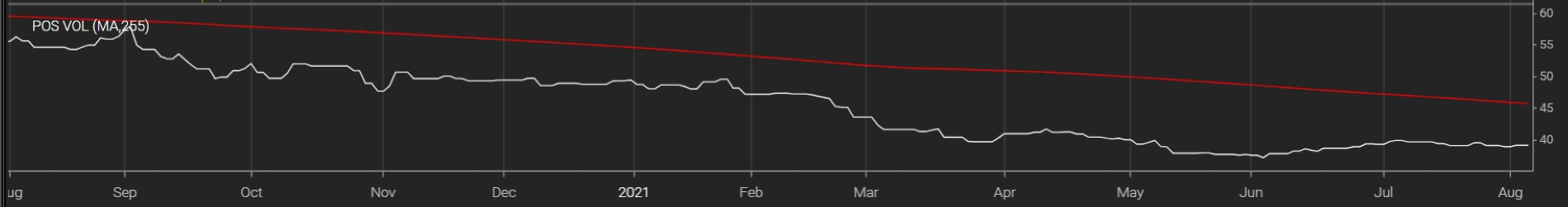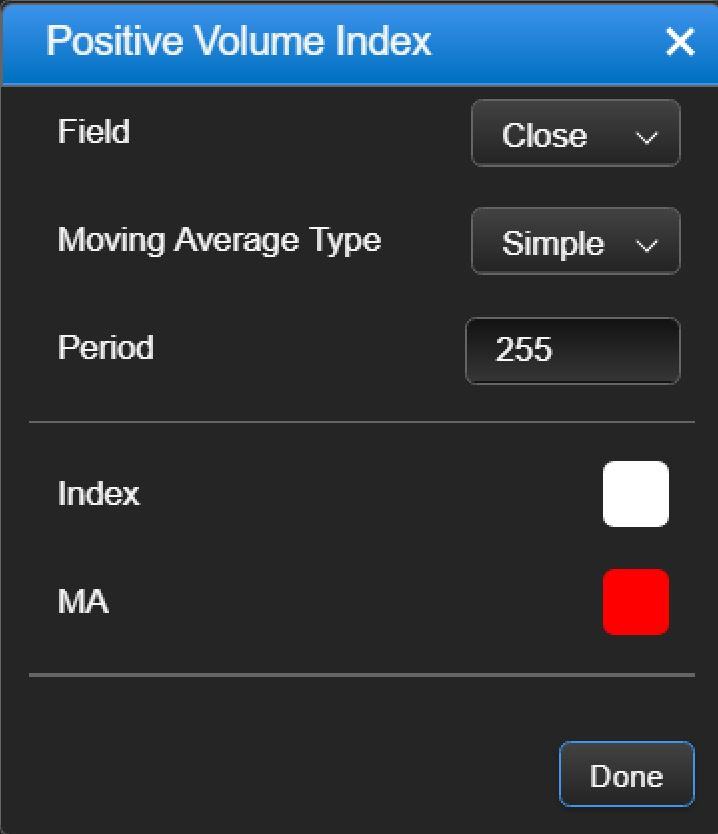# Positive Volume Index

#### ThinkStudy

##### New member
Anyway to do a Positive Volume Index on TOS that plots a moving average and has customizable inputs?[/IMG][/IMG]

Last edited by a moderator:
Solution

The final script with priceclose feild working:

# ########################################################
# Positive Volume Index
# ########################################################

declare lower;

input AvgType = averageType.SIMPLE;
input Length = 50;
input priceclose = close;

def index = compoundValue(1, index + if (volume > volume and priceclose != 0) then 100 * (priceclose - priceclose) / priceclose else 0, 100);

plot PVI = index;
plot AVG = MovingAverage(AvgType, index, Length);
PVI.SetDefaultColor(GetColor(5));
AVG.SetDefaultColor(color.yellow);
Here's what i got, but it doesn't work:

# Positive Volume Index

declare lower;

input AvgType = averageType.SIMPLE;
input Length = 50;
input priceclose = close;

def index = compoundValue(1, index + if (volume > volume and close != 0) then 100 * (close - close) / close else 0, 100);

plot PVI = index;
plot AVG = MovingAverage(AvgType, close, Length);
PVI.SetDefaultColor(GetColor(5));
AVG.SetDefaultColor(color.yellow);

Last edited by a moderator:
im not too concerned about having ALL the Moving Average Type input options of Simple, Exponential, Double Exponential, Triple Exponential, Hull, Time Series, Triangular, Variable, VIDYA, Weighted and Welles Wilder. I jsut mainly want a moving average with the Field Inputs of Open, High, Low, Close, hl/2, hl/3, hlcc/4 and ohlc/4 , and the period length input.

Last edited by a moderator:
plot AVG = MovingAverage(AvgType, close, Length);
You want an average of `index` instead of an average of `close`.

•ThinkStudy
You want an average of `index` instead of an average of `close`.
Thank You! That did the trick.

# ########################################################
# Positive Volume Index
# ########################################################

declare lower;

input AvgType = averageType.SIMPLE;
input Length = 50;
input priceclose = close;

def index = compoundValue(1, index + if (volume > volume and close != 0) then 100 * (close - close) / close else 0, 100);

plot PVI = index;
plot AVG = MovingAverage(AvgType, index, Length);
PVI.SetDefaultColor(GetColor(5));
AVG.SetDefaultColor(color.yellow);

You want an average of `index` instead of an average of `close`.
it doesnt look like the input priceclose feild is working, everything else is working on it though. do you know how to fix that?

it doesnt look like the input priceclose feild is working, everything else is working on it though. do you know how to fix that?
priceclose is not used anywhere in the code.

Do you need it to work? If you use open or high or low instead of close does this still give you a useful result? The built in PositiveVolumeIndex study has no option for price type. It always uses close. So I would just delete the `input priceclose = close;` line.

If you want it to work then everywhere on the line of `def index =` should be priceclose instead of close.

•ThinkStudy

Ruby:
``````# ########################################################
# Positive Volume Index
# ########################################################

declare lower;

input AvgType = averageType.SIMPLE;
input Length = 50;

def index = compoundValue(1, index + if (volume > volume and close != 0) then 100 * (close - close) / close else 0, 100);

plot PVI = index;
plot AVG = MovingAverage(AvgType, index, Length);
PVI.SetDefaultColor(GetColor(5));
AVG.SetDefaultColor(color.yellow);``````

The final script with priceclose feild working:

# ########################################################
# Positive Volume Index
# ########################################################

declare lower;

input AvgType = averageType.SIMPLE;
input Length = 50;
input priceclose = close;

def index = compoundValue(1, index + if (volume > volume and priceclose != 0) then 100 * (priceclose - priceclose) / priceclose else 0, 100);

plot PVI = index;
plot AVG = MovingAverage(AvgType, index, Length);
PVI.SetDefaultColor(GetColor(5));
AVG.SetDefaultColor(color.yellow);

Hi I've been trying to write code for this scanner. Specifically for when the 1. PVI has reversed direction and showing an inflection point positive . 2. This happens underneath the AVG line. 3. The PVI is not crossed up over the avg line 4. The average has a positive slope over the past 5 bars.

It runs but it gives mixed results and is driving me crazy!!!

script posvolume {
# Positive Volume Index
# ########################################################

# ########################################################
# Positive Volume Index
# ########################################################

declare lower;

input AvgType = averageType.SIMPLE;
input Length = 50;
input priceclose = close;

def index = compoundValue(1, index + if (volume > volume and priceclose != 0) then 100 * (priceclose - priceclose) / priceclose else 0, 100);

plot PVI = index;
plot AVG = MovingAverage(AvgType, index, Length);
PVI.SetDefaultColor(GetColor(5));
AVG.SetDefaultColor(color.yellow);

}

input AvgType = AverageType.EXPONENTIAL;
input Length = 30;
input priceclose = close;

def index = CompoundValue(1, index + if (volume > volume and priceclose != 0) then 100 * (priceclose - priceclose) / priceclose else 0, 100);

def PVI = index;
def AVG = MovingAverage(AvgType, index, Length);

def condition1 = Between(posvolume("length" = 30)."PVI", 0.99 * posvolume("length" = 30)."AVG", posvolume("length" = 30)."AVG") within 1 bar;
def condition2 = posvolume("length" = 30)."PVI" > posvolume("length" = 30)."PVI" within 1 bar;

def condition3 = PVI < AVG within 1 bar;

plot example = condition1 and condition2 and condition3 within 1 bar;

Hi I've been trying to write code for this scanner. Specifically for when the 1. AVG has reversed direction and showing an inflection point positive and positive slope for at least 2 bars. I also want to incorporate rsi and scan for when rsi is under the avg given the first condition is true. IS IT POSSIBLE TO REPLACE PVI WITH RSI ON THE STUDY???

THIS IS WHAT IM TALKING ABOUT VISUALLY FOR THE AVG LINE

input AvgType = AverageType.EXPONENTIAL;
input Length = 30;
input priceclose = close;

def index = CompoundValue(1, index + if (volume > volume and priceclose != 0) then 100 * (priceclose - priceclose) / priceclose else 0, 100);

def PVI = index;
def AVG = MovingAverage(AvgType, index, Length);

def condition2 = posvolume("length" = 30)."avg" > posvolume("length" = 30)."avg" within 1 bar;

def condition3 = RSI < AVG within 1 bar; (OBVIOUSLY I KNOW THIS WONT WORK BUT THIS IS THE CONDITION I WANT TO CODE FOR INCLUDED IN MY SCANNER AND STUDY)

plot example = condition2 and condition3 is true within 1 bar;

Last edited:
Hi I've been trying to write code for this scanner. Specifically for when the 1. avg has reversed direction and showing an inflection point positive and postive slope for at least 2 bars. I also want to incorporate rsi and scan for when rsi is under the avg given the first condition is true.

THIS IS WHAT IM TALKING ABOUT VISUALLY FOR THE AVG LINE
It runs but it gives mixed results and is driving me crazy!!!

script posvolume {
# Positive Volume Index
# ########################################################

# ########################################################
# Positive Volume Index
# ########################################################

declare lower;

input AvgType = averageType.SIMPLE;
input Length = 50;
input priceclose = close;

def index = compoundValue(1, index + if (volume > volume and priceclose != 0) then 100 * (priceclose - priceclose) / priceclose else 0, 100);

plot PVI = index;
plot AVG = MovingAverage(AvgType, index, Length);
PVI.SetDefaultColor(GetColor(5));
AVG.SetDefaultColor(color.yellow);

}

input AvgType = AverageType.EXPONENTIAL;
input Length = 30;
input priceclose = close;

def index = CompoundValue(1, index + if (volume > volume and priceclose != 0) then 100 * (priceclose - priceclose) / priceclose else 0, 100);

def PVI = index;
def AVG = MovingAverage(AvgType, index, Length);

def condition2 = posvolume("length" = 30)."avg" > posvolume("length" = 30)."avg" within 1 bar;

def condition3 = RSI < AVG within 1 bar;

plot example = condition2 and condition3 is true within 1 bar;

Hi Conmayne, you have two moving averages and the their inputs named the same, I believe they should be different, input and input1,....

Ruby:
``````# ########################################################
# Positive Volume Index
# ########################################################

declare lower;

input AvgType = averageType.SIMPLE;
input Length = 50;

def index = compoundValue(1, index + if (volume > volume and close != 0) then 100 * (close - close) / close else 0, 100);

plot PVI = index;
plot AVG = MovingAverage(AvgType, index, Length);
PVI.SetDefaultColor(GetColor(5));
AVG.SetDefaultColor(color.yellow);``````
Hi, I have a question, when the Volume goes below Moving average, does it mean the momentum is decreasing and Bears are getting nervous?

Hi Conmayne, you have two moving averages and the their inputs named the same, I believe they should be different, input and input1,....

I adjusted That was a typo and thinkscript bug it does when adding that code to the scanner. My intentions are positive reversal of the AVG vol line (yellow) and and rsi that is under the Yellow line on the same study. I have no use for the PVI line(red)

Hi I've been trying to write code for this scanner. Specifically for when the 1. AVG has reversed direction and showing an inflection point positive and positive slope for at least 2 bars. I also want to incorporate rsi and scan for when rsi is under the avg given the first condition is true. IS IT POSSIBLE TO REPLACE PVI WITH RSI ON THE STUDY???

THIS IS WHAT IM TALKING ABOUT VISUALLY FOR THE AVG LINE

input AvgType = AverageType.EXPONENTIAL;
input Length = 30;
input priceclose = close;

def index = CompoundValue(1, index + if (volume > volume and priceclose != 0) then 100 * (priceclose - priceclose) / priceclose else 0, 100);

def PVI = index;
def AVG = MovingAverage(AvgType, index, Length);

def condition2 = posvolume("length" = 30)."avg" > posvolume("length" = 30)."avg" within 1 bar;

def condition3 = RSI < AVG within 1 bar; (OBVIOUSLY I KNOW THIS WONT WORK BUT THIS IS THE CONDITION I WANT TO CODE FOR INCLUDED IN MY SCANNER AND STUDY)

plot example = condition2 and condition3 is true within 1 bar;

UPDATE:

Solved my first problem it seems with this code:

input AvgType = AverageType.EXPONENTIAL;
input Length = 30;
input priceclose = close;

def index = CompoundValue(1, index + if (volume > volume and priceclose != 0) then 100 * (priceclose - priceclose) / priceclose else 0, 100);

def PVI = index;
def AVG = MovingAverage(AvgType, index, Length);

#def condition1 = Between(posvolume("length" = 30)."PVI", 0.99 * posvolume("length" = 30)."AVG", posvolume("length" = 30)."AVG") within 1 bar;
#def condition2 = posvolume("length" = 30)."PVI" > posvolume("length" = 30)."PVI" within 1 bar;

def condition3 = Avg > avg and avg < avg;

plot scan = condition3 is true within 1 bars ;

NOW:

Im trying to figure out how to use rsi in place of PVI. PVI is needed for the calcualtion of AVG it seems so thats my dilemna.

### Not the exact question you're looking for?

87k+ Posts
209 Online## The Market Trading Game Changer

Join 2,500+ subscribers inside the useThinkScript VIP Membership Club
• Exclusive indicators
• Proven strategies & setups
• Private Discord community
• Exclusive members-only content
• 1 full year of unlimited support

What is useThinkScript?

useThinkScript is the #1 community of stock market investors using indicators and other tools to power their trading strategies. Traders of all skill levels use our forums to learn about scripting and indicators, help each other, and discover new ways to gain an edge in the markets.

How do I get started?

We get it. Our forum can be intimidating, if not overwhelming. With thousands of topics, tens of thousands of posts, our community has created an incredibly deep knowledge base for stock traders. No one can ever exhaust every resource provided on our site.

If you are new, or just looking for guidance, here are some helpful links to get you started.

What are the benefits of VIP Membership?